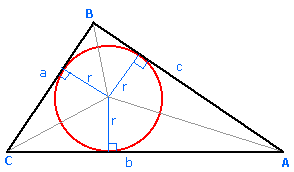# Use calculus, get thrilled !!

Calculus Level 2What is the $least$ $perimeter$ of an isosceles triangle in which a circle of radius $\sqrt{3}$ can be inscribed ?

Note : The picture shown is a rough one.

×